• +91 9971497814
• info@interviewmaterial.com

# RD Chapter 13- Linear Equations in Two Variables Ex-13.3 Interview Questions Answers

### Related Subjects

Question 1 :
Draw the graph of each of the following linear equations in two variables:
(i) x + y = 4 (ii) x – y = 2 (iii) -x + y = 6
(iv) y = 2x (v) 3x + 5y = 15 (vi) x/2 − y/3 = 2
(vii) (x−2)/3 = y – 3 (viii) 2y = -x +1

(i) Given : x + y = 4
or y = 4 – x,
Find values of x and y:
Putting x = 0 ⇒ y = 4
Putting x = 4 ⇒ y = 0
Graph:
Mark points (0, 4) and (4, 0) on the graph and join them.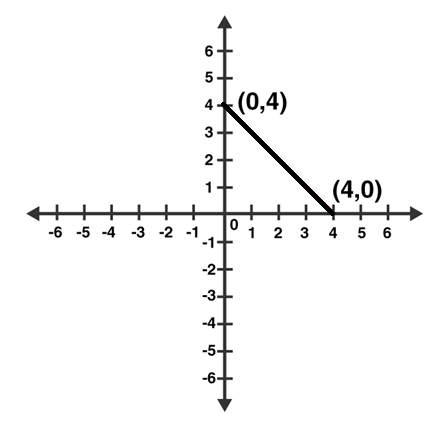(ii) Given: x – y = 2
So, y = x – 2
Putting x = 0 ⇒ y = – 2
Putting x = 2 ⇒ y = 0
Graph:
Mark points (0, -2) and (2, 0) on the graph and join them.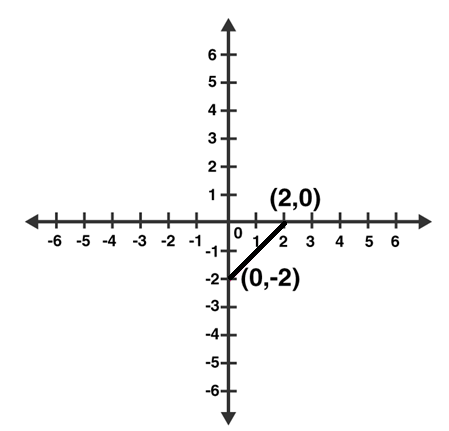(iii) Given: – x + y = 6
So, y = 6 + x
Putting x = 0 ⇒ y =6
Putting x = -6 ⇒ y = 0
Graph:
Mark points (0, 6) and (-6, 0) on the graph and join them.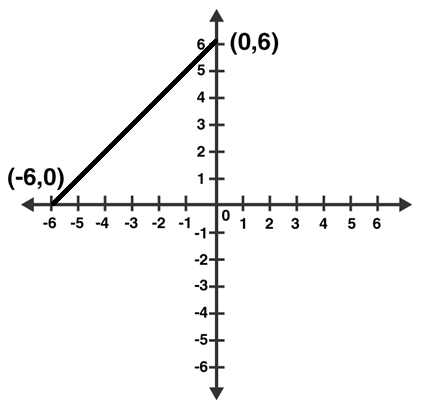(iv) Given: y = 2x
Put x = 1 ⇒ y = 2
Put x = 3 ⇒ y = 6
Graph:
Mark points (1, 2) and (3, 6) on the graph and join them.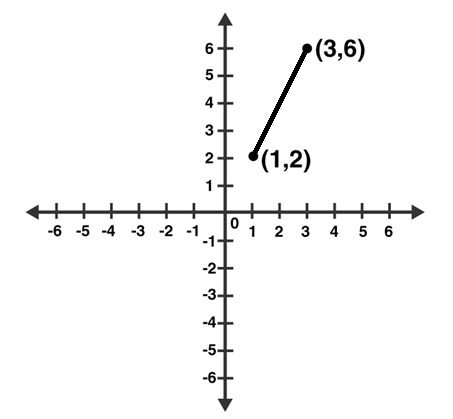(v) Given: 3x + 5y = 15
Or 5y = 15 – 3x
Putting x = 0 ⇒ 5y = 15 ⇒ y =3
Putting x = 5 ⇒ 5y = 0 ⇒ y = 0
Graph:
Mark points (0, 3) and (5, 0) on the graph and join them.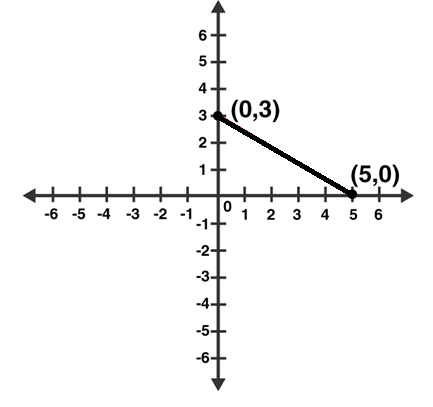(vi) Given: x/2 – y/3 = 2
3x – 2y = 12
y = (3x–12)/2
Putting x = 0 ⇒ y = -6
Putting x = 4 ⇒ y = 0
Graph:
Mark points (0, -6) and (4, 0) on the graph and join them.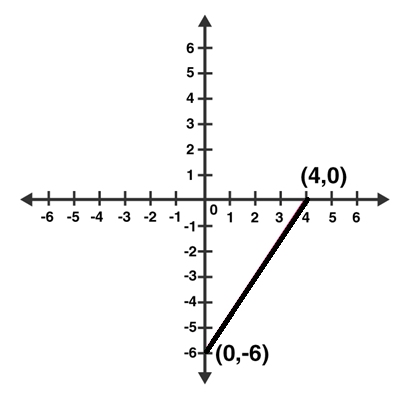(vii) Given: (x −2)/3 = y − 3
x – 2 = 3(y – 3)
x – 2 = 3y – 9
x = 3y – 7
Now, put x = 5 in x = 3y – 7
y = 4
Putting x = 8 in x = 3y – 7,
y = 5
Graph:
Mark points (5, 4) and (8, 5) on the graph and join them.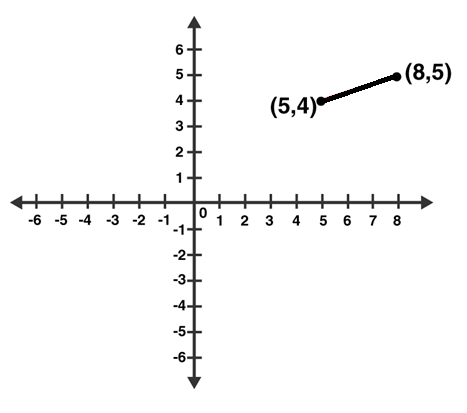(viii) Given: 2y = – x +1
2y = 1 – x
Now, putting x = 1 in 2y = 1 – x, we get;
y = 0
Again, putting x = 5 in 2y = 1 – x, we get;
y = -2
Graph:
Mark points (1, 0) and (5, -2) on the graph and join them.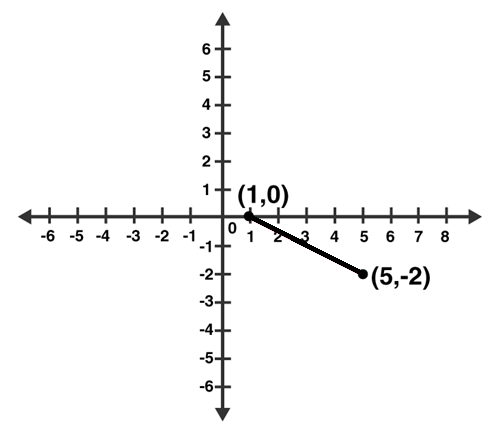Question 2 : Give the equations of two lines passing through (3, 12). How many more such lines are there, and why?

Since a = 3 and b = 12 is the solution of required equations. So we have to find the set of any two equations which satisfy this point.
Consider 4a – b = 0 and 3a – b + 3 = 0 set of lines which are passing through (3, 12).
We know, infinite lines can be pass through a point.
So, there are infinite lines passing through (3, 12).

Question 3 : A three-wheeler scooter charges Rs 15 for first kilometer and Rs 8 each for every subsequent kilometer. For a distance of x km, an amount of Rs y is paid. Write the linear equation representing the above information.

Let, total fare for covering the distance of ‘x’ km is given by Rs y
As per the given statement;
y = 15 + 8(x – 1)
y = 15 + 8x – 8
y = 8x + 7
Above equation represents the linear equation for the given information.

Question 4 : A lending library has a fixed charge for the first three days and an additional charge for each day thereafter. Aarushi paid Rs 27 for a book kept for seven days. If fixed charges are Rs x and per day charges are Rs y. Write the linear equation representing the above information.

Aarushi paid Rs 27, of which Rs. x for the first three days and Rs. y per day for 4 more days is given by
x + (7 – 3) y = 27
x + 4y = 27
Above equation represents the linear equation for the given information.

Question 5 : A number is 27 more than the number obtained by reversing its digits. lf its unit’s and ten’s digit are x and y respectively, write the linear equation representing the statement.

Given: The original number is 27 more than the number obtained by reversing its digits
The given number is in the form of 10y + x.
Number produced by reversing the digits of the number is 10x + y.
As per statement:
10y + x = 10x + y + 27
10y – y + x – 10x = 27
9y – 9x = 27
9 (y – x) = 27
y – x = 3
x – y + 3 = 0
Above equation represents the required linear equation.

Question 6 : The Sum of a two digit number and the number obtained by reversing the order of its digits is 121. If units and ten’s digit of the number are x and y respectively then write the linear equation representing the above statement.

As per the statement given, the number is 10y + x.
On reversing the digits of the number, we get, 10x + y
Sum of the two numbers is 121. (Given)
10y + x + 10x + y = 121
11x + 11y = 121
x + y = 11
Which represents the required linear equation.

Question 7 : Plot the Points (3, 5) and (-1, 3) on a graph paper and verify that the straight line passing through the points, also passes through the point (1, 4).

Plot points (3, 5), (-1, 3) and (1, 4) on a graph.
Let A(1, 4), B(3, 5) and C(-1, 3)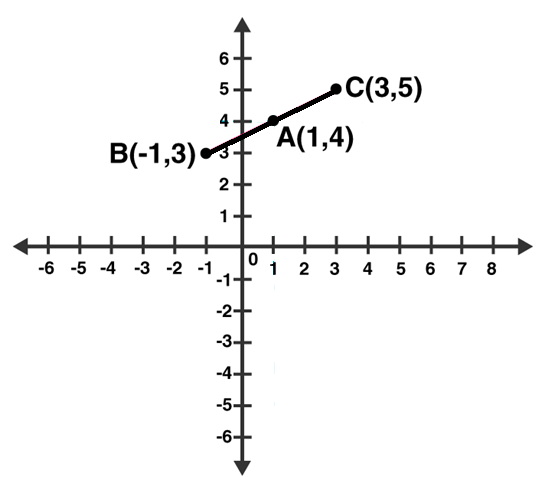From above graph, we can see that, Point A (1, 4) is already plotted on the graph, and a point of intersection of two intersecting lines.
Hence, it is proved that the straight line passing through (3, 5) and (-1, 3) and also passes through A (1, 4).

Question 8 :
From the choices given below, choose the equations whose graph is given in figure.
(i) y = x (ii) x + y = 0 (iii) y = 2x (iv) 2 + 3y = 7x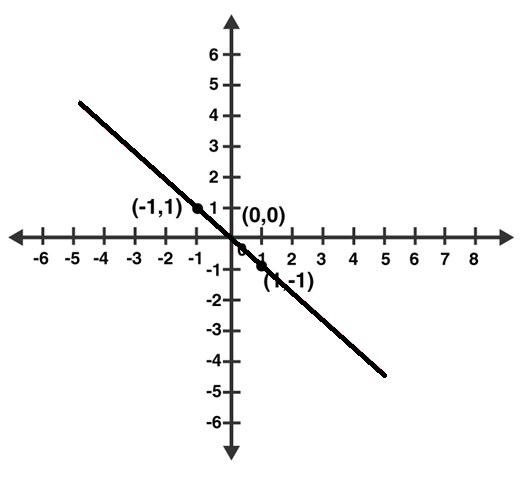From graph, co-ordinates (1, -1) and (-1, 1) are solutions of one of the equations.
We will put the value of all the co-ordinates in each equation and check which equation satisfy them.
(i) y = x
Put x = 1 and y = -1 ,
Thus, 1 ≠ -1
L.H.S ≠ R.H.S
Putting x = -1 and y = 1 ,
-1 ≠ 1
L.H.S ≠ R.H.S
Therefore, y = x does not represent the graph in the given figure.

(ii) x + y = 0
Putting x = 1 and y = -1 ,
⇒ 1 + (-1) = 0
⇒ 0 = 0
L.H.S = R.H.S
Putting x = -1 and y = 1 ,
(-1) + 1 = 0
0 = 0
L.H.S = R.H.S
Thus, the given solutions satisfy this equation.

(iii) y = 2x
Putting x = 1 and y = -1
-1 = 2 (Not True)
Putting x = -1 and y = 1
1 = -2 (Not True)
Thus, the given solutions does not satisfy this equation.

(iv) 2 + 3y = 7x
Putting x = 1 and y = -1
2 – 3 = 7
-1 = 7 (Not true)
Putting x = -1 and y = 1
2 + 3 = -7
5 = -7 (Not True)
Thus, the given solutions does not satisfy this equation.

Question 9 :
From the choices given below, choose the equation whose graph is given fig:
(i) y = x + 2 (ii) y = x – 2 (iii)y = – x + 2 (iv) x + 2y = 6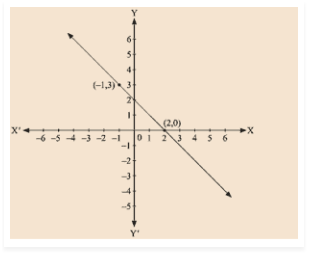Given: (-1, 3) and (2, 0) are the solution of one of the following given equations.
Check which equation satisfy both the points.
(i) y = x + 2
Putting, x = -1 and y = 3
3 ≠ – 1 + 2
L.H.S ≠ R.H.S
Putting, x = 2 and y = 0
0 ≠ 4
L.H.S ≠ R.H.S
Thus, this solution does not satisfy the given equation.

(ii) y = x – 2
Putting, x = -1 and y = 3
3 ≠ – 1 – 2
L.H.S ≠ R.H.S
Putting, x = 2 and y = 0
0 = 0
L.H.S = R.H.S
Thus, the given solutions does not satisfy this equation completely.

(iii) y = – x + 2
Putting, x = – 1 and y = 3
3 = – ( – 1 ) + 2
L.H.S = R.H.S
Putting x = 2 and y = 0
0 = -2 + 2
0 = 0
L.H.S = R.H.S
Therefore, (0, 2) and (-1,3) satisfy this equation.
Hence, this is the graph for equation y = -x + 2 .

(iv) x + 2y = 6
Putting, x = – 1 and y = 3
-1 + 2(3) = 6
-1 + 6 = 6
5 = 6
L.H.S ≠ R.H.S
Putting x = 2 and y = 0
2 + 2(0) = 6
2 = 6
L.H.S ≠ R.H.S
Thus, this solution does not satisfy the given equation.

Question 10 : If the point (2, -2) lies on the graph of linear equation, 5x + ky = 4, find the value of k.

Point (2,-2) lies on the given linear equation, which implies (2, -2) satisfy this equation 5x + ky = 4.
Now, putting x = 2 and y = -2 in 5x + ky = 4
5 × 2 + ( -2 ) k = 4
10 – 2k = 4
2k = 10 – 4
2k = 6
k = 6/2 = 3
The value of k is 3.

Todays Deals### RD Chapter 13- Linear Equations in Two Variables Ex-13.3 Contributorskrishan

Name:
Email:

# Latest News# 9000 interview questions in different categories